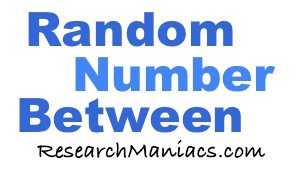Random Number Between 1 and 500Here is a random number between 1 and 500. You can press "Get Random Number" if you want to generate a different one.

When you ask to generate a random number between 1 and 500, grammatically speaking 1 and 500 should be excluded. However, we include 1 and 500 when generating a random number above because that is what most visitors expect it to do. You have to adjust the numbers above if you want to exclude them.

Random Numbers Between 1 and 501
Random Number Between 1 and 500 is not the only page we have! Go here for the next range we can generate random numbers for.